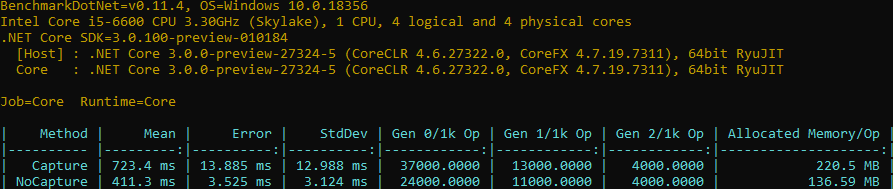# ConcurrentDictionary + closure = 💔

• Gérald Barré

In a previous post about performance tricks about strings, some of you mentioned they didn't know about the performance impact of captured variables in lambda expressions. So, let's see another example with the `ConcurrentDictionary<TKey, TValue>`.

The class `ConcurrentDictionary<TKey, TValue>` is often used for caching data, so you want it to be as fast as possible. In this usage, you mainly use 3 methods: `GetOrAdd`, `AddOrUpdate`, `TryGetValue`.

The second parameter of `GetOrAdd` and `AddOrUpdate` is often not use correctly. People often don't use the parameter of the delegate such as in the following code:

``````var dictionary = new ConcurrentDictionary<int, string>();
var key = 42;
dictionary.GetOrAdd(key, _ => key.ToString()); // Don't use this code``````

The problem here is that the lambda captures the variable `key`. In this case, the compiler will generate a new class and instantiate it just before calling `GetOrAdd`. This means your code will allocate, so more time spent to allocate the object and more time spent in the GC. Here's the code generated by the compiler:

``````public void Capture()
{
var concurrentDictionary = new ConcurrentDictionary<int, string>();
for (int key = 0; key < 1000000; ++key)
{
// instantiate the generated class
var cDisplayClass00 = new <>c__DisplayClass0_0();
cDisplayClass00.j = key;
concurrentDictionary.GetOrAdd(key, new Func<int, string>((object) cDisplayClass00, __methodptr(<Capture>b__0)));
}
}``````

Instead you should use the parameter of the delegate:

``````var dictionary = new ConcurrentDictionary<int, string>();
var key = 42;

In this case there is no captured variable, so the code generated by the compiler is more optimized. Indeed, the compiler still generates a class, but it uses a singleton to refer.

``````public void NoCapture()
{
var concurrentDictionary = new ConcurrentDictionary<int, string>();
for (int key = 0; key < 1000000; ++key)
{
concurrentDictionary.GetOrAdd(key, <>c.<>9__1_0 ?? (<>c.<>9__1_0 = new Func<int, string>((object) <>c.<>9, __methodptr(<NoCapture>b__1_0))));
}
}

[CompilerGenerated]
[Serializable]
private sealed class <>c
{
public static Func<int, string> <>9__1_0;

static <>c()
{
<>c.<>9 = new <>c();
}

internal string <NoCapture>b__1_0(int key)
{
return key.ToString();
}
}``````

## #Performance

Using BenchmarDotNet, you can compare the performance of each implementation:

``````internal static class Program
{
private static void Main() => BenchmarkRunner.Run<Benchmark>();
}

[CoreJob]
[MemoryDiagnoser]
public class Benchmark
{
[Benchmark]
public void Capture()
{
var dictionary = new ConcurrentDictionary<int, string>();
for (int i = 0; i < 1000000; i++)
{
var j = i; // Ensure we capture one variable per iteration
}
}

[Benchmark]
public void NoCapture()
{
var dictionary = new ConcurrentDictionary<int, string>();
for (int i = 0; i < 1000000; i++)
{